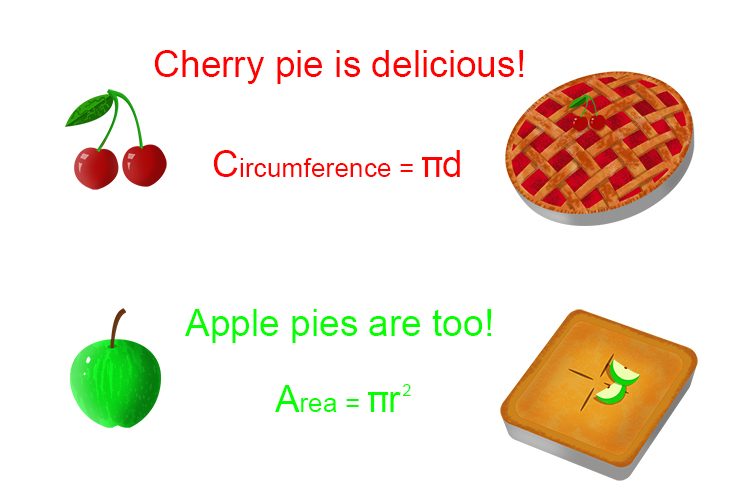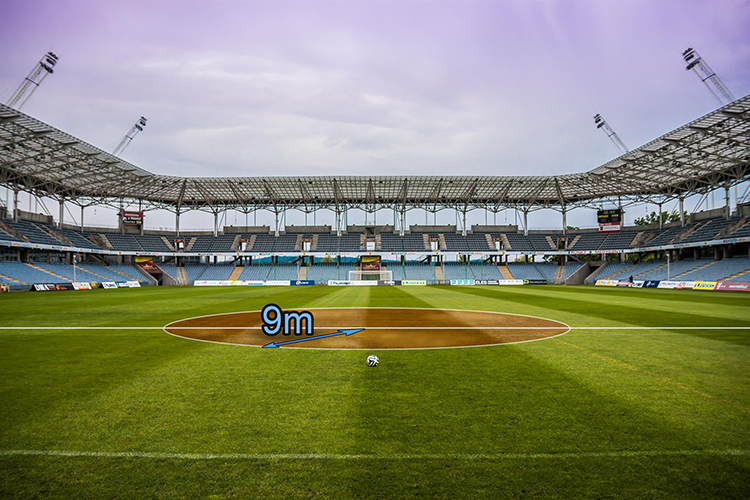# Remember the circumference and area of a circle formula

The circumference of a circle =\pi\timesdiameter

The area of a circle                  =\pi\times\radius\times\radius

Or =\pi\times\radius^2

So to remember these formula the following will help:NOTE:

Circumference=\pi\times\diameter

Is the same as   Circumference=2\pir

And then            Area=\pir^2

Example 1

The grass in the centre circle of a school’s football pitch has died. The grounds keeper has seen they can buy a pack of grass seed that will cover an area of 150m2 but if the centre circle has a radius of 9 metres will one pack be enough?The area of a circle = \pir^2

Therefore the area of the centre circle =\pi\times\9^2=\pi\times81=254.5m^2

One pack of grass seed will not be enough to reseed the centre circle of the football pitch.

Example 2

A council contractor has been asked to paint 20 mini roundabouts each with a diameter of 4m. The purchasing manager at the contractors contacts 3 suppliers to check prices stock levels and coverage per tin of paint as shown in the table below. From which supplier should the contractor purchase the paint?

 Supplier Blimey No Quality Boys & Boden Terribly Pricey Cost per tin £5.00 £5.30 £5.10 Coverage per tin 10m2 10m2 8m2 Tins in stock 20 35 35

Stage 1 - First work out the area to be covered by all the mini roundabouts

The area of a circle = \pir^2

Radius = half diameter so each mini roundabout has a radius = 4÷2 = 2m

Therefore area of each mini roundabout = \pi\times2^2 = 12.57m^2

Total area of all roundabouts = 20times12.57=251.40m^2

Stage 2 – Now work out how many tins would be required from each supplier to paint all the mini roundabouts.

Blimey No Quality

251.4/10=25.14  Therefore 26 tins would be required

So this supplier does not have enough tins in stock to paint all the mini roundabouts.

Boys & Boden

251.4/10=25.14  Therefore 26 tins would be required

Terribly Pricey

251.4/8=231.43  Therefore 32 tins would be required

Stage 3 – Work out the cost for the required number of paint tins from the suppliers.

Boys & Boden

26times£5.30=£137.80

Terribly Pricey

32times£5.10=£163.20